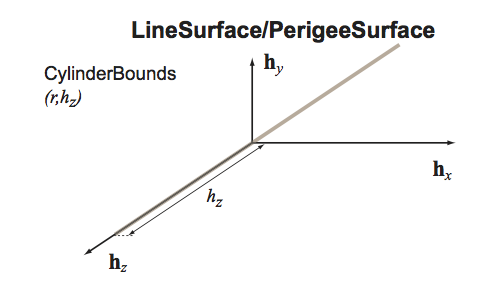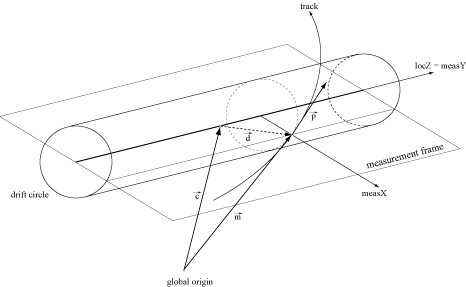# Class Acts::LineSurface

class LineSurface : public Acts::Surface

Base class for a linear surfaces in the TrackingGeometry to describe dirft tube, straw like detectors or the Perigee It inherits from Surface.Note

It leaves the type() method virtual, so it can not be instantiated

Subclassed by Acts::PerigeeSurface, Acts::StrawSurface

Public Functions

LineSurface() = delete
~LineSurface() override = default
virtual AlignmentToPathMatrix alignmentToPathDerivative(const GeometryContext &gctx, const FreeVector &parameters) const final

Calculate the derivative of path length at the geometry constraint or point-of-closest-approach w.r.t.

alignment parameters of the surface (i.e. local frame origin in global 3D Cartesian coordinates and its rotation represented with extrinsic Euler angles)

Parameters
• gctx – The current geometry context object, e.g. alignment

• parameters – is the free parameters

Returns

Derivative of path length w.r.t. the alignment parameters

virtual Vector3 binningPosition(const GeometryContext &gctx, BinningValue bValue) const final

The binning position is the position calculated for a certain binning type.

Parameters
• gctx – The current geometry context object, e.g. alignment

• bValue – is the binning type to be used

Returns

position that can beused for this binning

virtual const SurfaceBounds &bounds() const final

This method returns the bounds of the surface by reference.

virtual BoundToFreeMatrix boundToFreeJacobian(const GeometryContext &gctx, const BoundVector &boundParams) const final

Calculate the jacobian from local to global which the surface knows best, hence the calculation is done here.

Parameters
• gctx – The current geometry context object, e.g. alignment

• boundParams – is the bound parameters vector

Returns

Jacobian from local to global

virtual FreeToPathMatrix freeToPathDerivative(const GeometryContext &gctx, const FreeVector &parameters) const final

Calculate the derivative of path length at the geometry constraint or point-of-closest-approach w.r.t.

free parameters

Parameters
• gctx – The current geometry context object, e.g. alignment

• parameters – is the free parameters

Returns

Derivative of path length w.r.t. free parameters

virtual Result<Vector2> globalToLocal(const GeometryContext &gctx, const Vector3 &position, const Vector3 &direction, double tolerance = s_onSurfaceTolerance) const final

Specified for LineSurface: global to local method without dynamic memory allocation.

This method is the true global -> local transformation. It makes use of globalToLocal and indicates the sign of the Acts::eBoundLoc0 by the given momentum direction.

The calculation of the sign of the radius (or $$d_0$$) can be done as follows: May $$\vec d = \vec m - \vec c$$ denote the difference between the center of the line and the global position of the measurement/predicted state. Then, $$\vec d$$ lies in the so-called measurement plane. The latter is determined by the two orthogonal vectors $$\vec{\texttt{measY}} = \vec{e}_z$$ and $$\vec{\texttt{measX}} = \vec{\texttt{measY}} \times \frac{\vec{p}}{|\vec{p}|}$$.

The sign of the radius (or $$d_{0}$$ ) is then defined by the projection of $$\vec{d}$$ on $$\vec{measX}$$:$$sign = -sign(\vec{d} \cdot \vec{measX})$$Parameters
• gctx – The current geometry context object, e.g. alignment

• position – global 3D position - considered to be on surface but not inside bounds (check is done)

• direction – global 3D momentum direction (optionally ignored)

• tolerance – (unused)

Returns

A Result<Vector2>, which is set to !ok() if the position is not the point of closest approach to the line surface.

virtual SurfaceMultiIntersection intersect(const GeometryContext &gctx, const Vector3 &position, const Vector3 &direction, const BoundaryCheck &bcheck = false, ActsScalar tolerance = s_onSurfaceTolerance) const final

Calculate the straight-line intersection with the line surface.

Mathematical motivation:

Given two lines in parameteric form:

$$\vec l_{a}(u) = \vec m_a + u \cdot \vec e_{a}$$

$$\vec l_{b}(\mu) = \vec m_b + \mu \cdot \vec e_{b}$$

The vector between any two points on the two lines is given by:

$$\vec s(u, \mu) = \vec l_{b} - l_{a} = \vec m_{ab} + \mu \cdot \vec e_{b} - u \cdot \vec e_{a}$$,

where $$\vec m_{ab} = \vec m_{b} - \vec m_{a}$$.

$$\vec s(u_0, \mu_0)$$ denotes the vector between the two closest points

$$\vec l_{a,0} = l_{a}(u_0)$$ and $$\vec l_{b,0} = l_{b}(\mu_0)$$

and is perpendicular to both, $$\vec e_{a}$$ and $$\vec e_{b}$$.

This results in a system of two linear equations:

• (i) $$0 = \vec s(u_0, \mu_0) \cdot \vec e_a = \vec m_{ab} \cdot \vec e_a + \mu_0 \vec e_a \cdot \vec e_b - u_0$$

• (ii) $$0 = \vec s(u_0, \mu_0) \cdot \vec e_b = \vec m_{ab} \cdot \vec e_b + \mu_0 - u_0 \vec e_b \cdot \vec e_a$$

Solving (i) and (ii) for $$u$$ and $$\mu_0$$ yields:

• $$u_0 = \frac{(\vec m_{ab} \cdot \vec e_a)-(\vec m_{ab} \cdot \vec e_b)(\vec e_a \cdot \vec e_b)}{1-(\vec e_a \cdot \vec e_b)^2}$$

• $$\mu_0 = - \frac{(\vec m_{ab} \cdot \vec e_b)-(\vec m_{ab} \cdot \vec e_a)(\vec e_a \cdot \vec e_b)}{1-(\vec e_a \cdot \vec e_b)^2}$$

The function checks if $$u_0 \simeq 0$$ to check if the current position is at the point of closest approach, i.e. the intersection point, in which case it will return an onSurace intersection result. Otherwise, the path length from position to the point of closest approach ( $$u_0$$) is returned in a reachable intersection.

Note

expected to be normalized

Parameters
• gctx – The current geometry context object, e.g. alignment

• position – The global position as a starting point

• direction – The global direction at the starting point

• bcheck – The boundary check directive for the estimate

• tolerance – the tolerance used for the intersection

Returns

is the intersection object

Vector3 lineDirection(const GeometryContext &gctx) const
virtual ActsMatrix<2, 3> localCartesianToBoundLocalDerivative(const GeometryContext &gctx, const Vector3 &position) const final

Calculate the derivative of bound track parameters local position w.r.t.

position in local 3D Cartesian coordinates

Parameters
• gctx – The current geometry context object, e.g. alignment

• position – The position of the parameters in global

Returns

Derivative of bound local position w.r.t. position in local 3D cartesian coordinates

virtual Vector3 localToGlobal(const GeometryContext &gctx, const Vector2 &lposition, const Vector3 &direction) const final

Local to global transformation.

Note

for line surfaces the momentum direction is used in order to interpret the drift radius

Parameters
• gctx – The current geometry context object, e.g. alignment

• lposition – is the local position to be transformed

• direction – is the global momentum direction (used to sign the closest approach)

Returns

global position by value

virtual std::string name() const override

Return properly formatted class name for screen output.

inline Vector3 normal(const GeometryContext &gctx) const

Normal vector return without argument.

virtual Vector3 normal(const GeometryContext &gctx, const Vector2 &lposition) const final

The normal vector is undefined if we do not know the momentum.

Parameters
• gctx – The current geometry context object, e.g. alignment

• lposition – is the local position is ignored

Returns

a zero vector

Vector3 normal(const GeometryContext &gctx, const Vector2 &lposition) const = 0

Normal vector return without argument.

Vector3 normal(const GeometryContext &gctx, const Vector3 &position) const

Normal vector return without argument.

LineSurface &operator=(const LineSurface &other)

Assignment operator.

Parameters

other – is the source surface dor copying

virtual double pathCorrection(const GeometryContext &gctx, const Vector3 &position, const Vector3 &direction) const override

the pathCorrection for derived classes with thickness is by definition 1 for LineSurfaces

Note

input parameters are ignored

Note

there’s no material associated to the line surface

virtual RotationMatrix3 referenceFrame(const GeometryContext &gctx, const Vector3 &position, const Vector3 &direction) const final

Return the measurement frame - this is needed for alignment, in particular.

for StraightLine and Perigee Surface

• the default implementation is the RotationMatrix3 of the transform

Parameters
• gctx – The current geometry context object, e.g. alignment

• position – is the global position where the measurement frame is constructed

• direction – is the momentum direction used for the measurement frame construction

Returns

is a rotation matrix that indicates the measurement frame